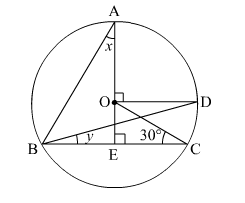# In the given figure, O is the centre of the circle and ∠BCO = 30°. Find x and y.

Question:

In the given figure, O is the centre of the circle and ∠BCO = 30°. Find x and y.Solution:

In the given figure, OD is parallel to BC.

∴ ∠BCO = ∠COD    (Alternate interior angles)

$\Rightarrow \angle C O D=30^{\circ}$

We know that the angle subtended by an arc of a circle at the centre is double the angle subtended by it on the remaining part  of the circle.

Here, arc CD subtends ∠COD at the centre and ∠CBD at B on the circle.

∴ ∠COD = 2∠CBD

$\Rightarrow \angle C B D=\frac{30^{\circ}}{2}=15^{\circ}$    (from (1))

$\therefore y=15^{\circ}$            ...(2)

Also, arc AD subtends ∠AOD at the centre and ∠ABD at B on the circle.

∴ ∠AOD = 2∠ABD

$\Rightarrow \angle A B D=\frac{90^{\circ}}{2}=45^{\circ}$        ...(3)

In ∆ABE,
x + y + ∠ABD + ∠AEB = 180       (Sum of the angles of a triangle)
⇒  x + 15 + 45 + 90 = 180        (from (2) and (3))
⇒  x = 180 − (90+ 15 + 45)
⇒  x = 180 − 150
⇒  x = 30

Hence, x = 30 and y = 15.